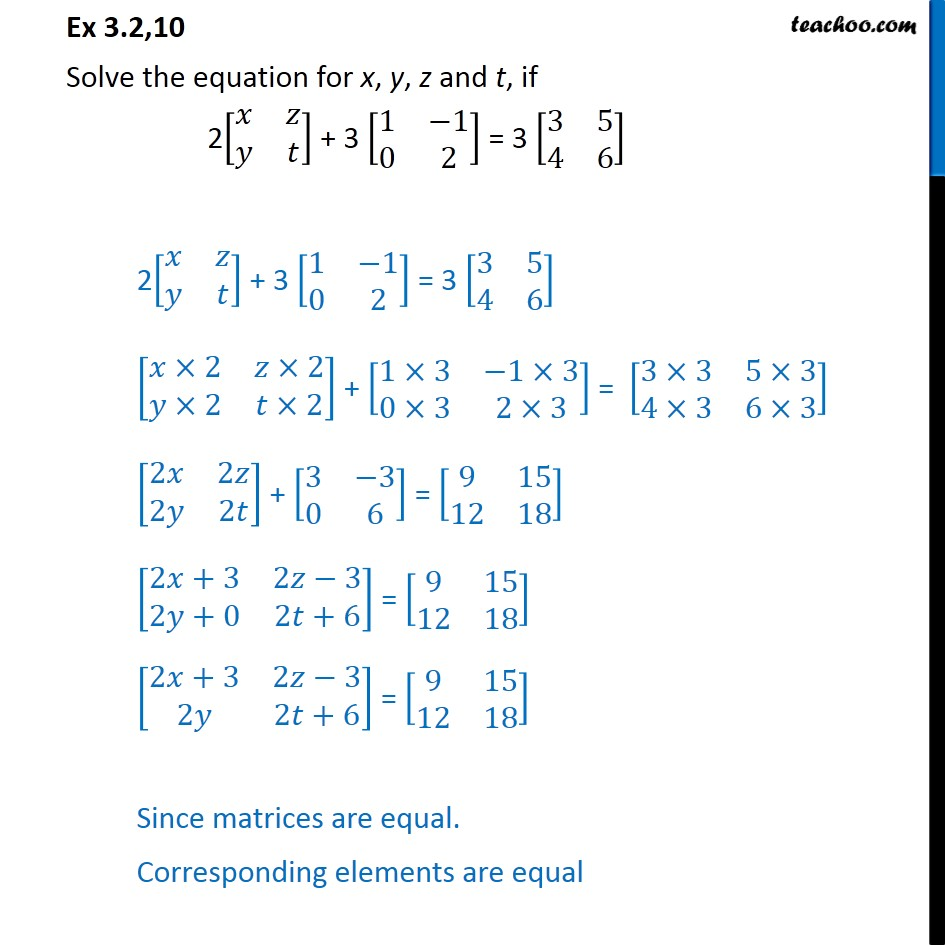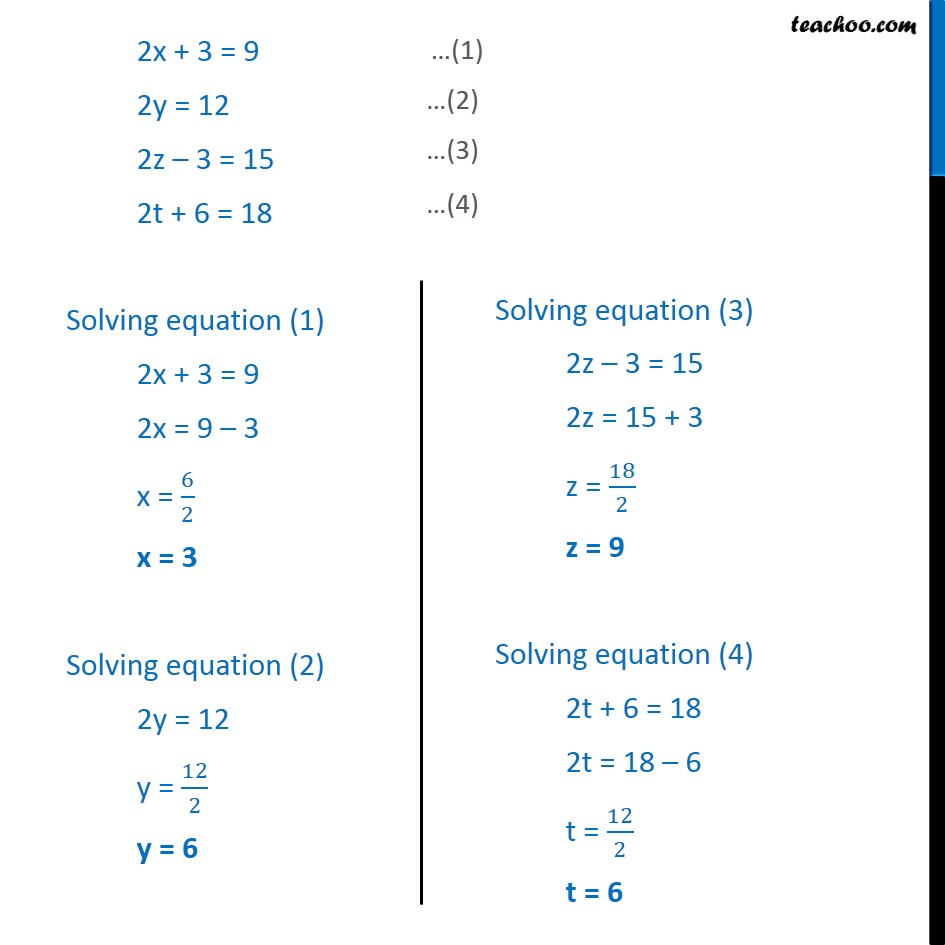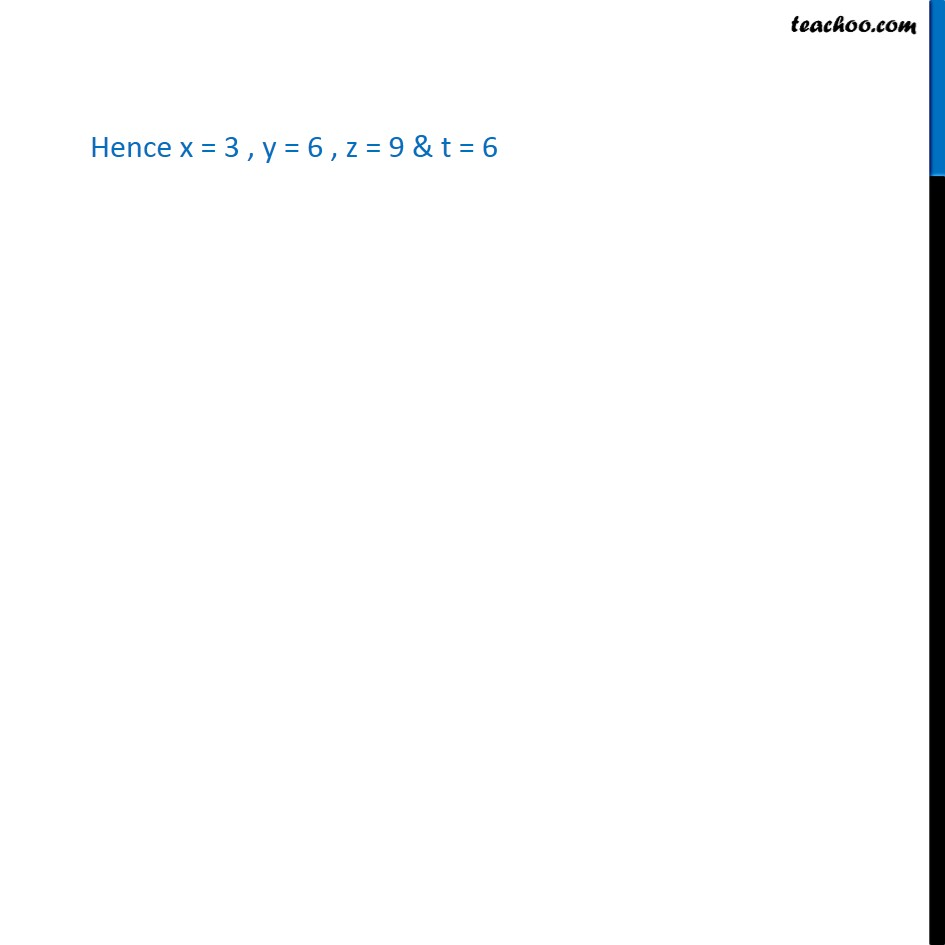Learn All Concepts of Chapter 3 Class 12 Matrices - FREE. Check - Matrices Class 12 - Full video1. Chapter 3 Class 12 Matrices
2. Serial order wise
3. Ex 3.2

Transcript

Ex 3.2,10 Solve the equation for x, y, z and t, if 2[■8(𝑥&𝑧@𝑦&𝑡)] + 3 [■8(1&−1@0&2)] = 3 [■8(3&5@4&6)] 2[■8(𝑥&𝑧@𝑦&𝑡)] + 3 [■8(1&−1@0&2)] = 3 [■8(3&5@4&6)] [■8(𝑥×2&𝑧×2@𝑦×2&𝑡×2)] + [■8(1×3&−1×3@0×3&2×3)] = [■8(3×3&5×3@4×3&6×3)] [■8(2𝑥&2𝑧@2𝑦&2𝑡)] + [■8(3&−3@0&6)] = [■8(9&15@12&18)] [■8(2𝑥+3&2𝑧−3@2𝑦+0&2𝑡+6)] = [■8(9&15@12&18)] [■8(2𝑥+3&2𝑧−3@2𝑦&2𝑡+6)] = [■8(9&15@12&18)] Since matrices are equal. Corresponding elements are equal 2x + 3 = 9 2y = 12 2z – 3 = 15 2t + 6 = 18 Solving equation (1) 2x + 3 = 9 2x = 9 – 3 x = 6/2 x = 3 Solving equation (2) 2y = 12 y = 12/2 y = 6 Hence x = 3 , y = 6 , z = 9 & t = 6

Ex 3.2

Chapter 3 Class 12 Matrices
Serial order wise